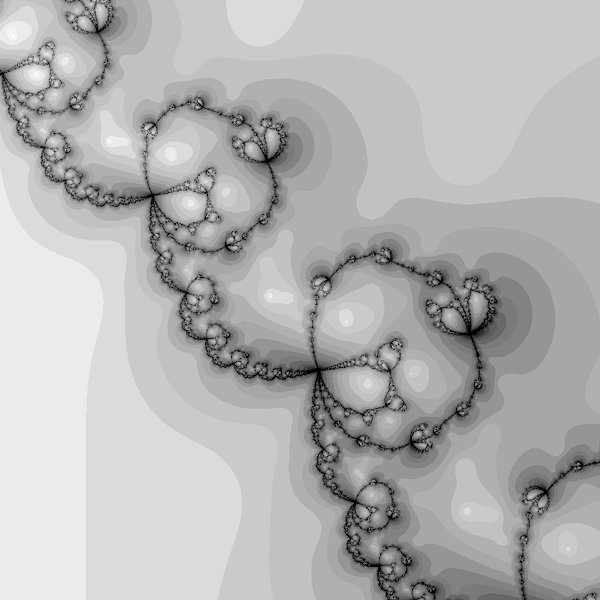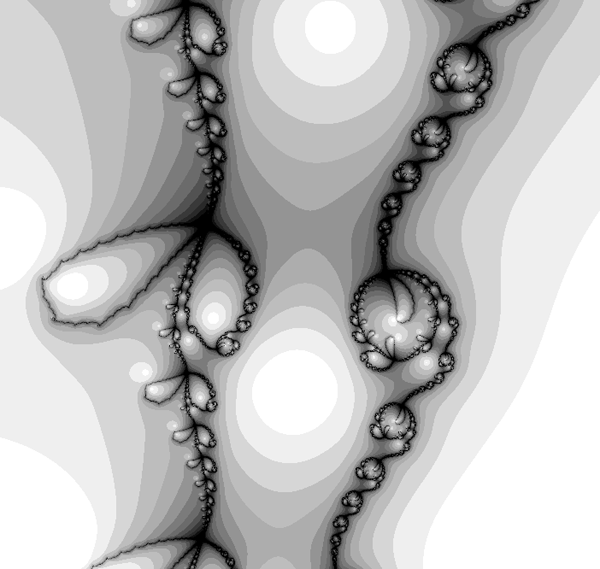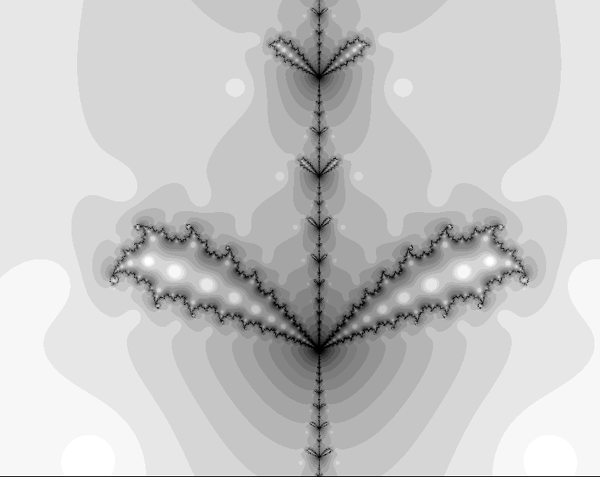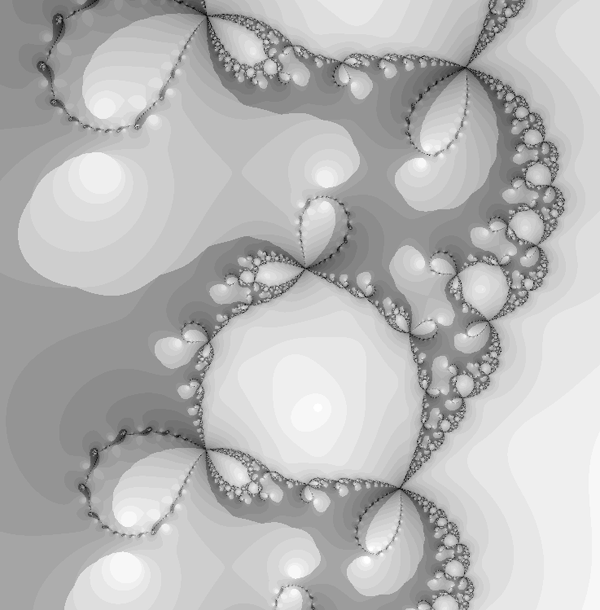# Newton Raphson Fractals

Written by Paul Bourke
Original August 1989, Updated April 2019

This fractal image technique is based on the Newton Raphson method of finding the solution (roots) to a polynomial equation of the form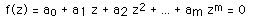The method generates a series where the n+1'th approximation to the solution is given by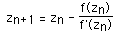where f'(zn) is the slope (first derivative) of f(z) evaluated at zn. To create a 2D image using this technique each point in a partition of the complex plane is used as initial guess, zo, to the solution. The point is coloured depending on which solution is found and/or how long it took to arrive at the solution. A simple example is an application of the above to find the three roots of the polynomial

z3 - 1 = 0

The following shows the three roots (red, green, blue circles) on the complex image plane and the grey level illustrates how long it takes the series to converge to one of those roots.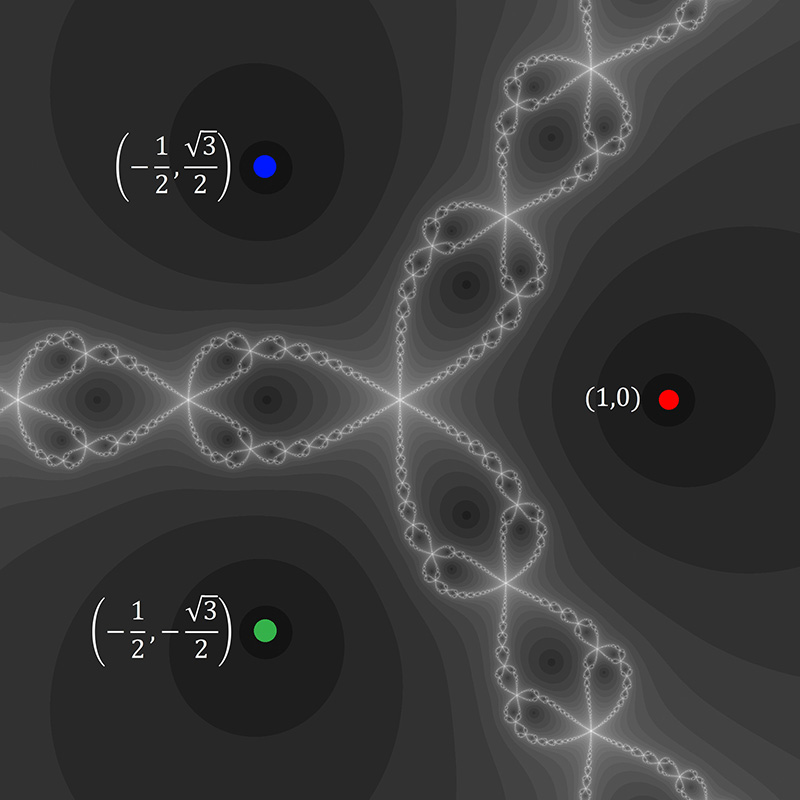A trademark of chaotic systems is that very similar initial conditions can give rise to very different behaviour. In the image above there are points very close together one of which converges to a root very quickly and the other converges very slowly.

To illustrate this further, it is not just the convergence rate that is sensitive to inital conditions but also the root actually converged to. The following colours the regions where points converge to one of the three roots, shaded as red, green and blue. Again, points arbitrarily close to each other can converge to different roots.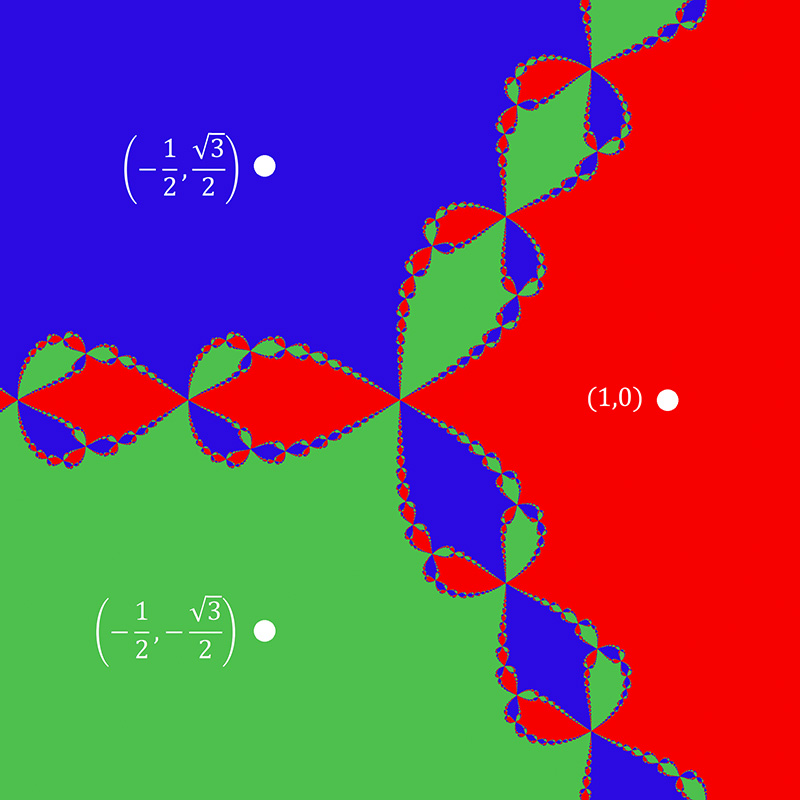Examples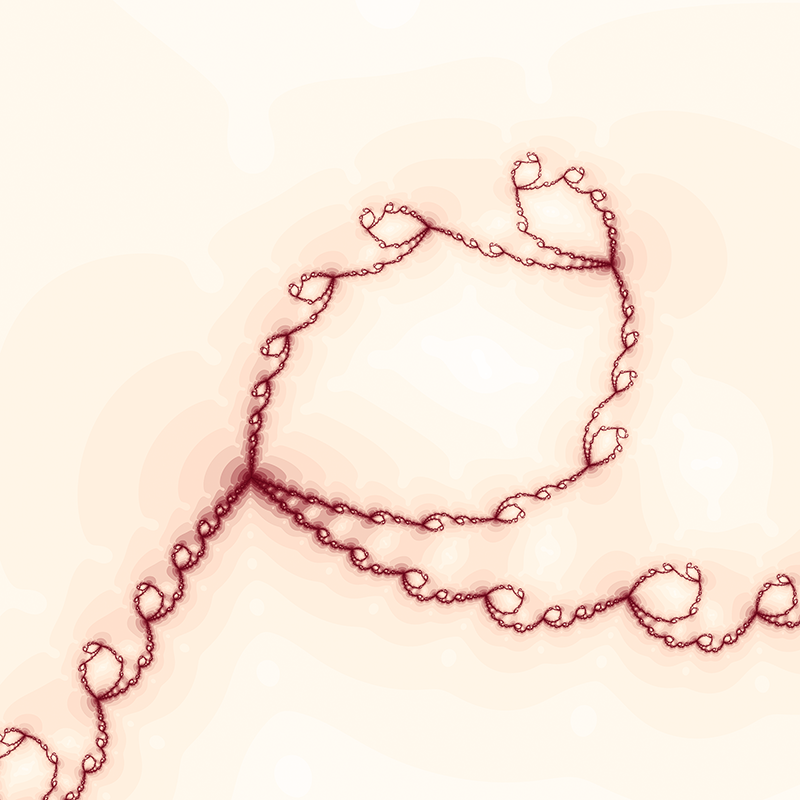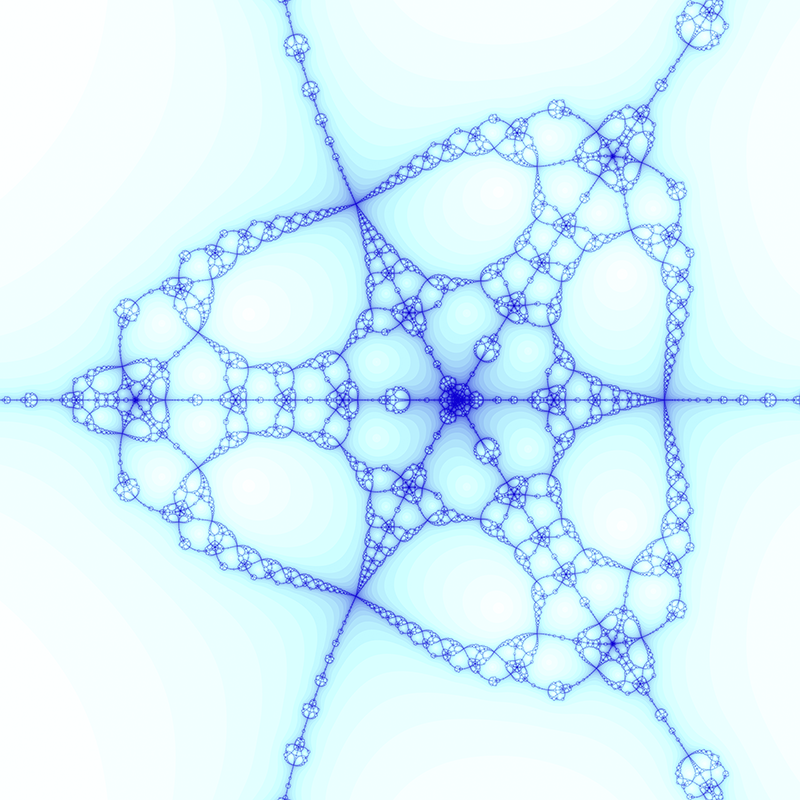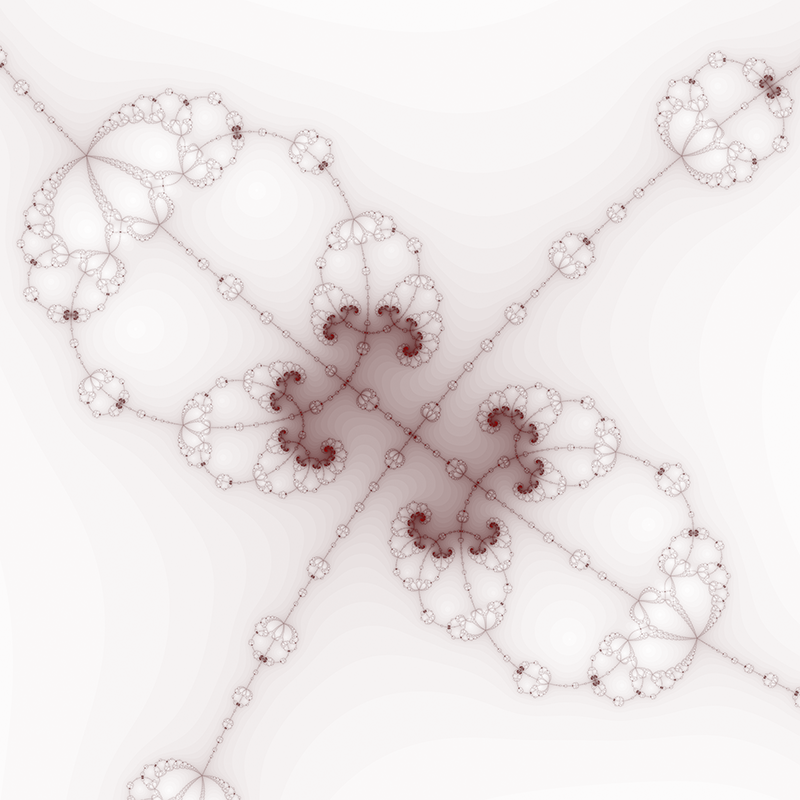Older examples from 1989